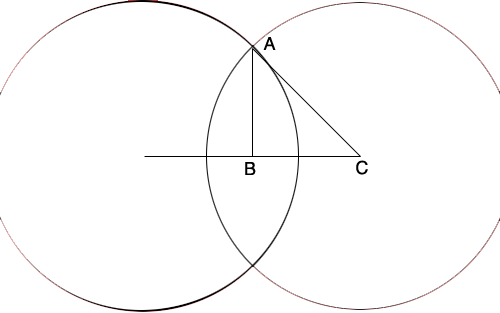SEARCH HOMEMath Central Quandaries & QueriesQuestion from Husen, a student: two circles of radius 5 cm intersect each other .the distance between their centers is 5root 2.find the area of the portion common to the two circlesHusen,

I can help get you started.C is the centre of the circle on the right and B is the midpoint of the line joining the centres. By the symmetry angle ABC is a right angle. The length of CA is 5 cm and the length of BC is 1/2 (5√2) cm. Use Pythagoras theorem to find the length of AB. What is the measure of the angle BCA.

PennyMath Central is supported by the University of Regina and The Pacific Institute for the Mathematical Sciences.